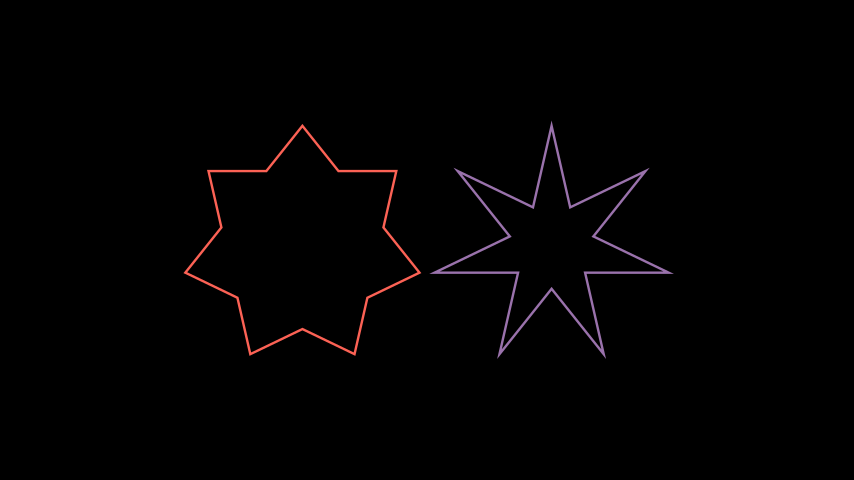# Star#

Qualified name: `manim.mobject.geometry.polygram.Star`

Bases: `Polygon`

A regular polygram without the intersecting lines.

Parameters:
• n (int) – How many points on the `Star`.

• outer_radius (float) – The radius of the circle that the outer vertices are placed on.

• inner_radius (float | None) –

The radius of the circle that the inner vertices are placed on.

If unspecified, the inner radius will be calculated such that the edges of the `Star` perfectly follow the edges of its `RegularPolygram` counterpart.

• density (int) –

The density of the `Star`. Only used if `inner_radius` is unspecified.

See `RegularPolygram` for more information.

• start_angle (float | None) – The angle the vertices start at; the rotation of the `Star`.

• kwargs – Forwardeds to the parent constructor.

Raises:

ValueError – If `inner_radius` is unspecified and `density` is not in the range `[1, n/2)`.

Examples

Example: StarExample```from manim import *

class StarExample(Scene):
def construct(self):

self.play(Create(star), run_time=3)
```
```class StarExample(Scene):
def construct(self):

self.play(Create(star), run_time=3)

```

Example: DifferentDensitiesExample```from manim import *

class DifferentDensitiesExample(Scene):
def construct(self):
density_2 = Star(7, outer_radius=2, density=2, color=RED)
density_3 = Star(7, outer_radius=2, density=3, color=PURPLE)

```
```class DifferentDensitiesExample(Scene):
def construct(self):
density_2 = Star(7, outer_radius=2, density=2, color=RED)
density_3 = Star(7, outer_radius=2, density=3, color=PURPLE)

```

Methods

Attributes

 `animate` Used to animate the application of any method of `self`. `animation_overrides` `color` `depth` The depth of the mobject. `fill_color` If there are multiple colors (for gradient) this returns the first one `height` The height of the mobject. `n_points_per_curve` `sheen_factor` `stroke_color` `width` The width of the mobject.

Initialize self. See help(type(self)) for accurate signature.

Parameters:
• n (int) –